# Calculated Members

Calculated members are members of a dimension or a measure group that are defined based on a combination of cube data, arithmetic operators, numbers, and functions. For example, you can create a calculated member that calculates the sum of two physical measures inthe cube. Calculated member definitions are stored in cubes, but their values are calculated at query time.

Switch to the Calculations tab of the Sales cube to start creating new Calculated Members.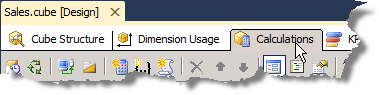### Profit

The calculated member we want to create in this cube is a Profit calculated member which is based on the already existing Amount and Cost measures.

First, click the New Calculated Member button on the calculations tab of the Sales cube design window.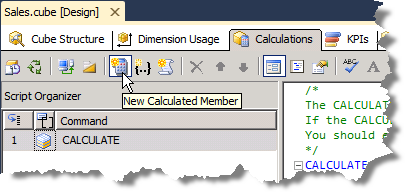First you name the new Calculated Member, e.g. ‘Profit’. (If the name contains spaces you must enclose it in square brackets, e.g. [Budget Amount]).

Then you must type in the expression that constitutes what we want to achieve with this new Calculated Member. However, instead of typing, it is both easier and safer to drag in the measures upon which the Calculated Member must be based.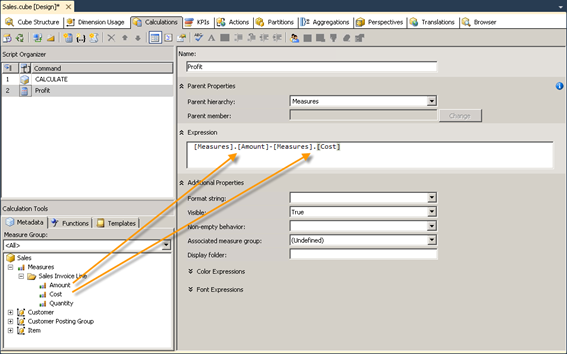### Number formatting of measures and calculated members

You can define the standard formatting of measures and calculated members as they should appear to end-users in e.g. a TARGIT client.

For measures this is done at the cube’s Cube structure tab where you can change the FormatString property for each measure.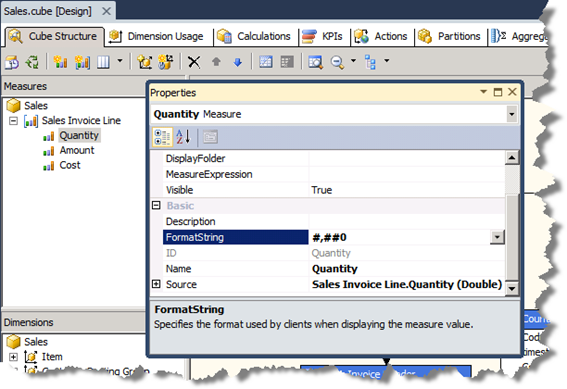Examples:

• #,##0 Thousands separator, at least one digit, no decimals.
• #.00 No thousands separator, two decimals.
• #0.0”%” At least one digit, one decimal, trailing text: %

Setting the format string for a calculated member is done at the cube’s Calculations tab where the format property is part of the general definition of the calculated member.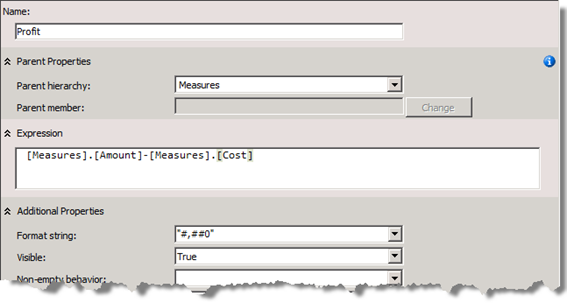Notice: Contrary to the format string of measures, format strings for calculated members must be enclosed in quotation marks, e.g. “#,##0”.